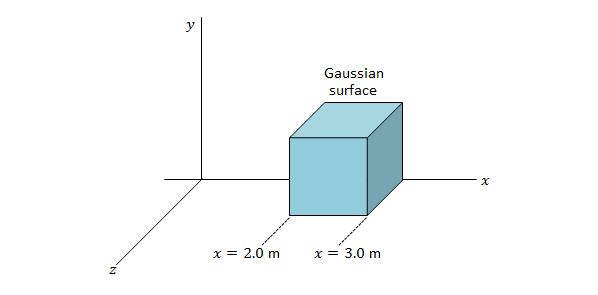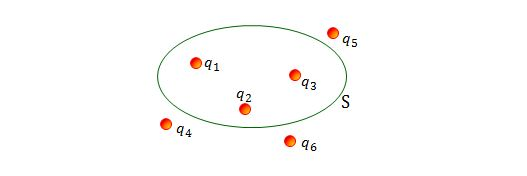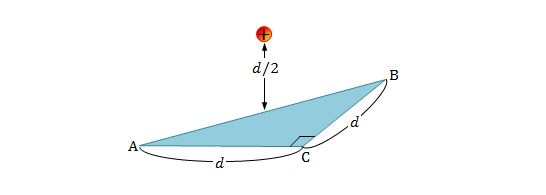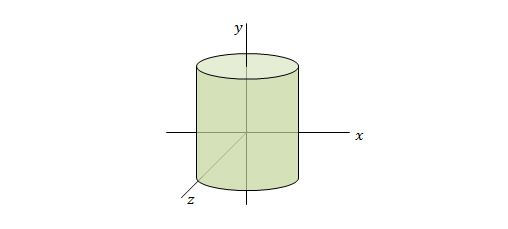Electricity and Magnetism

Gauss' lawA Gaussian cube of edge length $1.0\text{ m}$ is located in the $xyz$-space, as shown in the above figure. If the electric field in this space is expressed as $\overrightarrow{E}=5.0x\hat{i}+4.0\hat{j} \text{ N/C},$ what is the net charge enclosed by the Gaussian cube?

The value of the permittivity constant is $\varepsilon_0=8.85 \times 10^{-12} \text{ C}^2\text{/N}\cdot\text{m}^2.$

The electric field in a certain device is vertically downward. At a height of $27\text{ m}$ from the bottom of the device the magnitude of electric field is $50.0 \text{ N/C},$ and at a height of $18\text{ m}$ from the bottom the magnitude of electric field is $80.0\text{ N/C}.$ If the bottom face of a cube with side length $9\text{ m}$ is at a height of $18\text{ m}$ from the bottom of the device, what is the approximate net amount of charge contained in the cube?

The value of the permittivity constant is $\varepsilon_0=8.85 \times 10^{-12} \text{ C}^2\text{/N}\cdot\text{m}^2.$The above figure shows six charged particles, where three of them are enclosed by a Gaussian surface $S.$ The cross section of a $3$-D Gaussian surface $S$ is indicated as green ellipse. If $q_1=q_4=+3.9\text{ nC},$ $q_2=q_5=-5.3\text{ nC},$ and $q_3=q_6=-3.9\text{ nC},$ what is the net electric flux through the Gaussian surface?

The value of the permittivity constant is $\varepsilon_0=8.85 \times 10^{-12} \text{ C}^2\text{/N}\cdot\text{m}^2.$Consider an isosceles right triangle $ABC$ of base $d,$ as shown in the above figure. A proton is at a distance of $\frac{d}{2}$ directly above the midpoint of the hypotenuse $\overline{AB}.$ What is the magnitude of the electric flux through the triangle?

The charge of a proton is $q=+1.6 \times 10^{-19}\text{ C}$ and the value of the permittivity constant is $\varepsilon_0=8.85 \times 10^{-12} \text{ C}^2\text{/N}\cdot\text{m}^2.$Consider a cylinder base which is perpendicular to the $y$-axis, as shown in the above figure. At each point on the surface of the cylinder, the electric field is parallel to the $y$-axis. The area of the base of the cylinder is $9.0 \text{ m}^2$ and the height of the cylinder is $5.0 \text{ m}.$ On the top face of the cylinder the field is $\overrightarrow{E}=-42.0 \hat{j}\text{ N/C},$ and on the bottom face it is $\overrightarrow{E}=+22.0 \hat{j}\text{ N/C}.$ What is the approximate net charge contained within the cylinder?

The value of the permittivity constant is $\varepsilon_0=8.85 \times 10^{-12} \text{ C}^2\text{/N}\cdot\text{m}^2.$

×